Practice the questions of McGraw Hill Math Grade 4 Answer Key PDF Chapter 11 Test to secure good marks & knowledge in the exams.

Multiply to find each missing number.

Question 1.
3 kg = _____________ g
3000 g

Explanation:
1 kg = 1000 g
3 kg = 3 x 1000 = 3000 g
So, 3 kg = 3000 g.

Question 2.
3 lb = ____________ oz
1 lb = 16 oz
3 lb = 3 x 16 = 48 lb
So, 3 lb = 48 oz.

Question 3.
106 L = ______________ mL
106,000 ml

Explanation:
1 L = 1000 ml
106 L = 106 x 1000 = 106,000 ml
So, 106 L = 106,000 ml.

Question 4.
5 gal = _____________ pt
40 pt

Explanation:
1 gal = 8 pt
5 gal = 5 x 8 = 40 pt
So, 5 gal = 40 pt.

Question 5.
5 pt = _____________ c
10 c

Explanation:
1 pt = 2 c
5 pt = 5 x 2 = 10 c
So, 5 pt = 10 c.

Question 6.
5 minutes = __________ seconds
300 seconds

Explanation:
1 minute = 60 seconds
5 minutes = 5 x 60 = 300 seconds
So, 5 minutes = 300 seconds.

Place a check mark next to the best answer.

Question 7.
What is the mass of a small dog?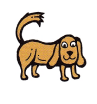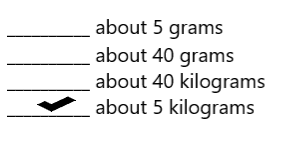Explanation:
The mass of a small dog is about 5 kilograms.

Question 8.
What does a school bus weigh?Explanation:
A school bus weigh about 10 tons.

Question 9.
What is the capacity of a can of soup?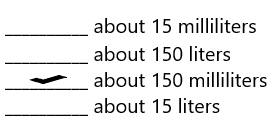Explanation:
The capacity of a can of soup is about 150 milliliters.

Question 10.
What is the capacity of a can of paint?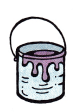Explanation:
The capacity of a an of paint is about 3 quarts.

Question 11.
How long does it take to eat lunch?Explanation:
It takes about 20 minutes to eat lunch.

The line plot shows the volume of water in liters in nine small fish tanks.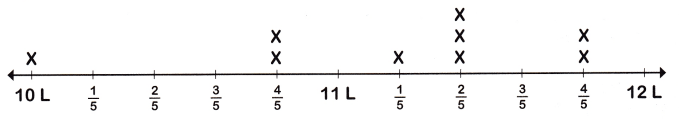Use the data in the line plot to answer questions 12-17.

Question 12.
What is the greatest volume shown on the line plot?
11$$\frac{4}{5}$$

Explanation:
The last X marks in above the line plot are at 11$$\frac{4}{5}$$
So, 11$$\frac{4}{5}$$ is the greatest volume shown on the line plot.

Question 13.
What is the most common volume shown on the line plot?
11$$\frac{2}{5}$$

Explanation:
There are more X marks above the fraction 11$$\frac{2}{5}$$
So, 11$$\frac{2}{5}$$ is the most common volume shown on the line plot.

Question 14.
How many fish tanks have a volume of 11$$\frac{1}{5}$$ liters?
1

Explanation:
In the above line plot only 1 x mark is marked against the fraction 11$$\frac{1}{5}$$
So, 1 fish tank have a volume of 11$$\frac{1}{5}$$ liters.

Question 15.
Which volume is an outlier?
10 L

Explanation:
10 L is far from the other numbers in the data set
It is an outliner
An outliner is any number that is very different from the rest of the numbers in the set
So, 10 L is an outliner.

Question 16.
What is the difference between the greatest volume and the least volume shown on the line plot?
1$$\frac{4}{5}$$

Explanation:
11$$\frac{4}{5}$$ is the greatest volume shown on the line plot
10 is the least volume shown on the line plot
Subtract to find
11$$\frac{4}{5}$$ – 10 = 1$$\frac{4}{5}$$ L
So, the difference between the greatest volume and the least volume shown on the line plot is 1$$\frac{4}{5}$$ L.

Question 17.
What is the sum of the capacities of the three smallest fish tanks?
30$$\frac{8}{5}$$

Explanation:
The capacities of the three smallest fish tanks are 10, 10$$\frac{4}{5}$$ and 10$$\frac{4}{5}$$
10 + 10$$\frac{4}{5}$$ +10$$\frac{4}{5}$$ = 30$$\frac{8}{5}$$ L
So, the sum of the capacities of the three smallest fish tanks is 30$$\frac{8}{5}$$ L.

Use the number line to solve.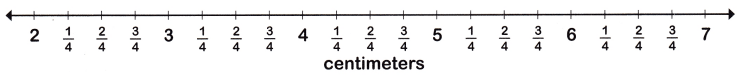Question 18.
Jenn measures some beetles she found in a garden. The first beetle is 4$$\frac{2}{4}$$ cm long. The second beetle is 1$$\frac{1}{4}$$ cm longer than the first beetle. How long is the second beetle?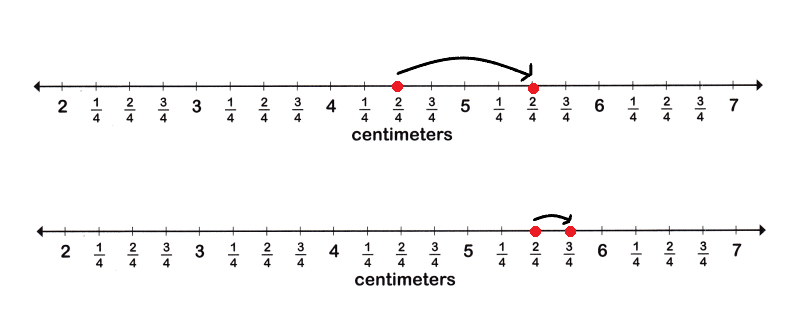Explanation:
Jenn measures some beetles she found in a garden
The first beetle is 4$$\frac{2}{4}$$ cm long
The second beetle is 1$$\frac{1}{4}$$ cm longer than the first beetle
I marked the numbers on the number line and drew jumps forward to add
4$$\frac{2}{4}$$ cm + 1$$\frac{1}{4}$$ cm = 5$$\frac{3}{4}$$ cm
So, the second bee is 5$$\frac{3}{4}$$ cm long.

Question 19.
A craft store sells buttons. Large black buttons are 5$$\frac{3}{4}$$ cm wide. Brown buttons are 3$$\frac{1}{4}$$ cm narrower than the black buttons. How wide are the brown buttons?Large black buttons are 5$$\frac{3}{4}$$ cm wide
Brown buttons are 3$$\frac{1}{4}$$ cm narrower than the black buttons
5$$\frac{3}{4}$$ cm – 3$$\frac{1}{4}$$ cm = 2$$\frac{2}{4}$$ cm
So, the brown buttons are 2$$\frac{2}{4}$$ cm long.